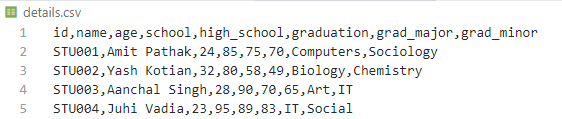GeeksforGeeks App
Open AppBrowser
Continue

# Convert nested JSON to CSV in Python

In this article, we will discuss how can we convert nested JSON to CSV in Python.

An example of a simple JSON file: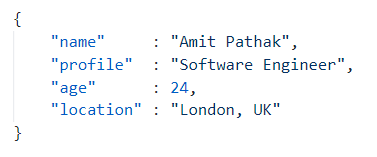A simple JSON representation

As you can see in the example, a single key-value pair is separated by a colon (:) whereas each key-value pairs are separated by a comma (,). Here, “name”, “profile”, “age”, and “location” are the key fields while the corresponding values are “Amit Pathak“, “Software Engineer“, “24”, “London, UK” respectively.

A nested JSON is a structure where the value for one or more fields can be an another JSON format. For example, follow the below example that we are going to use to convert to CSV format.

An example of a nested JSON file: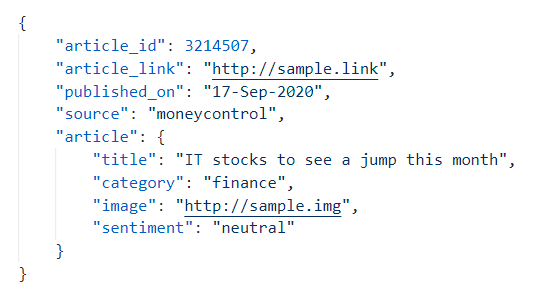A nested JSON example

In the above example, the key field “article” has a value which is another JSON format. JSON supports multiple nests to create complex JSON files if required.

## Nested JSON to CSV conversion

Our job is to convert the JSON file to a CSV format. There can be many reasons as to why we need to perform this conversion. CSV are easy to read when opened in a spreadsheet GUI application like Google Sheets or MS Excel. They are easy to work with for Data Analysis task. It is also a widely excepted format when working with tabular data since it is easy to view for humans, unlike the JSON format.

### Approach

• The first step is to read the JSON file as a python dict object. This will help us to make use of python dict methods to perform some operations. The read_json() function is used for the task, which taken the file path along with the extension as a parameter and returns the contents of the JSON file as a python dict object.
• We normalize the dict object using the normalize_json() function. It checks for the key-value pairs in the dict object. If the value is again a dict then it concatenates the key string with the key string of the nested dict.
• The desired CSV data is created using the generate_csv_data() function. This function concatenates each record using a comma (,) and then all these individual records are appended with a new line (‘\n’ in python).
• In the final step, we write the CSV data generated in the earlier step to a preferred location provided through the filepath parameter.

File used: article.json file

```{
"article_id": 3214507,
"published_on": "17-Sep-2020",
"source": "moneycontrol",
"article": {
"title": "IT stocks to see a jump this month",
"category": "finance",
"image": "http://sample.img",
"sentiment": "neutral"
}
}```

Example: Converting JSON to CSV

## Python

 `import` `json`` ` ` ` `def` `read_json(filename: ``str``) ``-``> ``dict``:`` ` `    ``try``:``        ``with ``open``(filename, ``"r"``) as f:``            ``data ``=` `json.loads(f.read())``    ``except``:``        ``raise` `Exception(f``"Reading {filename} file encountered an error"``)`` ` `    ``return` `data`` ` ` ` `def` `normalize_json(data: ``dict``) ``-``> ``dict``:`` ` `    ``new_data ``=` `dict``()``    ``for` `key, value ``in` `data.items():``        ``if` `not` `isinstance``(value, ``dict``):``            ``new_data[key] ``=` `value``        ``else``:``            ``for` `k, v ``in` `value.items():``                ``new_data[key ``+` `"_"` `+` `k] ``=` `v`` ` `    ``return` `new_data`` ` ` ` `def` `generate_csv_data(data: ``dict``) ``-``> ``str``:`` ` `    ``# Defining CSV columns in a list to maintain``    ``# the order``    ``csv_columns ``=` `data.keys()`` ` `    ``# Generate the first row of CSV ``    ``csv_data ``=` `","``.join(csv_columns) ``+` `"\n"`` ` `    ``# Generate the single record present``    ``new_row ``=` `list``()``    ``for` `col ``in` `csv_columns:``        ``new_row.append(``str``(data[col]))`` ` `    ``# Concatenate the record with the column information ``    ``# in CSV format``    ``csv_data ``+``=` `","``.join(new_row) ``+` `"\n"`` ` `    ``return` `csv_data`` ` ` ` `def` `write_to_file(data: ``str``, filepath: ``str``) ``-``> ``bool``:`` ` `    ``try``:``        ``with ``open``(filepath, ``"w+"``) as f:``            ``f.write(data)``    ``except``:``        ``raise` `Exception(f``"Saving data to {filepath} encountered an error"``)`` ` ` ` `def` `main():``    ``# Read the JSON file as python dictionary``    ``data ``=` `read_json(filename``=``"article.json"``)`` ` `    ``# Normalize the nested python dict``    ``new_data ``=` `normalize_json(data``=``data)`` ` `    ``# Pretty print the new dict object``    ``print``(``"New dict:"``, new_data)`` ` `    ``# Generate the desired CSV data ``    ``csv_data ``=` `generate_csv_data(data``=``new_data)`` ` `    ``# Save the generated CSV data to a CSV file``    ``write_to_file(data``=``csv_data, filepath``=``"data.csv"``)`` ` ` ` `if` `__name__ ``=``=` `'__main__'``:``    ``main()`

Output: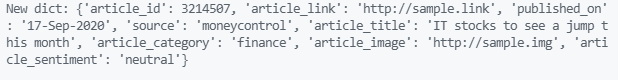Python console output for Code Block 1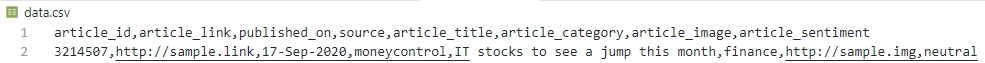CSV Output for Code Block 1

The same can be achieved through the use of Pandas Python library. Pandas is a free source python library used for data manipulation and analysis. It performs operations by converting the data into a pandas.DataFrame format. It offers a lot of functionalities and operations that can be performed on the dataframe.

### Approach

• The first step is to read the JSON file as a python dict object. This will help us to make use of python dict methods to perform some operations. The read_json() function is used for the task, which taken the file path along with the extension as a parameter and returns the contents of the JSON file as a python dict object.
• We normalize the dict object using the normalize_json() function. It check for the key-value pairs in the dict object. If the value is again a dict then it concatenates the key string with the key string of the nested dict.
• In this step, rather than putting manual effort for appending individual objects as each record of the CSV, we are using pandas.DataFrame() method. It takes in the dict object and generates the desired CSV data in the form of pandas DataFrame object. One thing in the above code is worth noting that, the values of the “new_data” dict variable are present in a list. The reason is that while passing a dictionary to create a pandas dataframe, the values of the dict must be a list of values where each value represents the value present in each row for that key or column name. Here, we have a single row.
• We use pandas.DataFrame.to_csv() method which takes in the path along with the filename where you want to save the CSV as input parameter and saves the generated CSV data in Step 3 as CSV.

Example: JSON to CSV conversion using Pandas

## Python

 `import` `json``import` `pandas`` ` ` ` `def` `read_json(filename: ``str``) ``-``> ``dict``:`` ` `    ``try``:``        ``with ``open``(filename, ``"r"``) as f:``            ``data ``=` `json.loads(f.read())``    ``except``:``        ``raise` `Exception(f``"Reading {filename} file encountered an error"``)`` ` `    ``return` `data`` ` ` ` `def` `normalize_json(data: ``dict``) ``-``> ``dict``:`` ` `    ``new_data ``=` `dict``()``    ``for` `key, value ``in` `data.items():``        ``if` `not` `isinstance``(value, ``dict``):``            ``new_data[key] ``=` `value``        ``else``:``            ``for` `k, v ``in` `value.items():``                ``new_data[key ``+` `"_"` `+` `k] ``=` `v``     ` `    ``return` `new_data`` ` ` ` `def` `main():``    ``# Read the JSON file as python dictionary``    ``data ``=` `read_json(filename``=``"article.json"``)`` ` `    ``# Normalize the nested python dict ``    ``new_data ``=` `normalize_json(data``=``data)`` ` `    ``print``(``"New dict:"``, new_data, ``"\n"``)`` ` `    ``# Create a pandas dataframe ``    ``dataframe ``=` `pandas.DataFrame(new_data, index``=``[``0``])`` ` `    ``# Write to a CSV file``    ``dataframe.to_csv(``"article.csv"``)`` ` ` ` `if` `__name__ ``=``=` `'__main__'``:``    ``main()`

Output: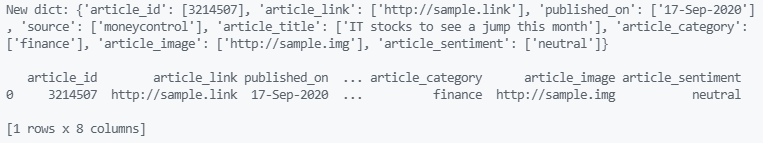python console output for Code Block 2CSV output for Code Block 2

The above two examples are good when we have a single level of nesting for JSON but as the nesting increases and there are more records, the above codes require more editing. We can handle such JSON with much ease using the pandas library. Let us see how.

## Convert N-nested JSON to CSV

Any number of nesting and records in a JSON can be handled with minimal code using “json_normalize()” method in pandas

Syntax:

json_normalize(data)

File in use: details.json file

```{
"details": [
{
"id": "STU001",
"name": "Amit Pathak",
"age": 24,
"results": {
"school": 85,
"high_school": 75,
},
"education": {
"major": "Computers",
"minor": "Sociology"
}
}
},
{
"id": "STU002",
"name": "Yash Kotian",
"age": 32,
"results": {
"school": 80,
"high_school": 58,
},
"education": {
"major": "Biology",
"minor": "Chemistry"
}
}
},
{
"id": "STU003",
"name": "Aanchal Singh",
"age": 28,
"results": {
"school": 90,
"high_school": 70,
},
"education": {
"major": "Art",
"minor": "IT"
}
}
},
{
"id": "STU004",
"age": 23,
"results": {
"school": 95,
"high_school": 89,
},
"education": {
"major": "IT",
"minor": "Social"
}
}
}
]
}```

Here the “details” key consists of an array of 4 elements, where each element contains 3-level of nested JSON objects. The “major” and “minor” key in each of these objects is in a level 3 nesting.

### Approach

• The first step is to read the JSON file as a python dict object. This will help us to make use of python dict methods to perform some operations. The read_json() function is used for the task, which taken the file path along with the extension as a parameter and returns the contents of the JSON file as a python dict object.
• We have iterated for each JSON object present in the details array. In each iteration we first normalized the JSON and created a temporary dataframe. This dataframe was then appended to the output dataframe.
• Once done, the column name was renamed for better visibility. If we see the console output, the “major” column was named as “education.graduation.major” before renaming. This is because the “json_normalize()” method uses the keys in the complete nest for generating the column name to avoid duplicate column issue. So, “education” is the first level, “graduation” is second and “major” is third level in the JSON nesting. Therefore, the column “education.graduation.major” was simply renamed to “graduation”.
• After renaming the columns, the to_csv() method saves the pandas dataframe object as CSV to the provided file location.

Example: Converting n-nested JSON to CSV

## Python

 `import` `json``import` `pandas`` ` ` ` `def` `read_json(filename: ``str``) ``-``> ``dict``:`` ` `    ``try``:``        ``with ``open``(filename, ``"r"``) as f:``            ``data ``=` `json.loads(f.read())``    ``except``:``        ``raise` `Exception(f``"Reading {filename} file encountered an error"``)`` ` `    ``return` `data`` ` ` ` `def` `create_dataframe(data: ``list``) ``-``> pandas.DataFrame:`` ` `    ``# Declare an empty dataframe to append records``    ``dataframe ``=` `pandas.DataFrame()`` ` `    ``# Looping through each record``    ``for` `d ``in` `data:``         ` `        ``# Normalize the column levels``        ``record ``=` `pandas.json_normalize(d)``         ` `        ``# Append it to the dataframe ``        ``dataframe ``=` `dataframe.append(record, ignore_index``=``True``)`` ` `    ``return` `dataframe`` ` ` ` `def` `main():``    ``# Read the JSON file as python dictionary ``    ``data ``=` `read_json(filename``=``"details.json"``)`` ` `    ``# Generate the dataframe for the array items in ``    ``# details key ``    ``dataframe ``=` `create_dataframe(data``=``data[``'details'``])`` ` `    ``# Renaming columns of the dataframe ``    ``print``(``"Normalized Columns:"``, dataframe.columns.to_list())`` ` `    ``dataframe.rename(columns``=``{``        ``"results.school"``: ``"school"``,``        ``"results.high_school"``: ``"high_school"``,``        ``"results.graduation"``: ``"graduation"``,``        ``"education.graduation.major"``: ``"grad_major"``,``        ``"education.graduation.minor"``: ``"grad_minor"``    ``}, inplace``=``True``)`` ` `    ``print``(``"Renamed Columns:"``, dataframe.columns.to_list())`` ` `    ``# Convert dataframe to CSV``    ``dataframe.to_csv(``"details.csv"``, index``=``False``)`` ` ` ` `if` `__name__ ``=``=` `'__main__'``:``    ``main()`

Output:

\$ Console Output

—–